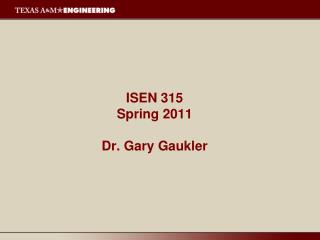Download PresentationISEN 315 Spring 2011 Dr. Gary Gaukler

# ISEN 315 Spring 2011 Dr. Gary Gaukler - PowerPoint PPT PresentationDownload Presentation## ISEN 315 Spring 2011 Dr. Gary Gaukler

- - - - - - - - - - - - - - - - - - - - - - - - - - - E N D - - - - - - - - - - - - - - - - - - - - - - - - - - -
##### Presentation Transcript

1. ISEN 315Spring 2011Dr. Gary Gaukler

2. Inventory Control • Deterministic inventory control • Stochastic inventory control • MRP / Lot sizing / JIT • Supply chain management

3. Reasons for Holding Inventories

4. Relevant Costs • Holding Costs - Costs proportional to the quantity of inventory held.

5. Relevant Costs (continued) • Ordering Cost (or Production Cost). Can include both fixed and variable components. slope = c K

6. Relevant Costs (continued) • Penalty or Shortage Costs. All costs that accrue when insufficient stock is available to meet demand.

7. Simple EOQ Model • Assumptions: 1. Demand is fixed at l units per unit time. 2. Shortages are not allowed. 3. Orders are received instantaneously. 4. Order quantity is fixed at Q per cycle. 5. Cost structure: a) Fixed and marginal order costs (K + cx) b) Holding cost at h per unit held per unittime.

8. Inventory Levels for the EOQ Model

9. Cost Equation for the EOQ Model

10. The Average Annual Cost Function G(Q)

11. The Average Annual Cost Function G(Q)

12. Properties of the EOQ Solution

13. Example • Desk production rate = 200 per month • Each desk needs 40 screws • Screws cost \$0.03 • Fixed delivery charges are \$100 per order • 25% interest rate for holding cost • What is the optimal order size?

14. Example

15. EOQ Cost Function

16. Quantity Discount Models • All Units Discounts: the discount is applied to ALL of the units in the order. • Incremental Discounts: the discount is applied only to the number of units above the breakpoint.

17. All-Units Discount Order Cost Function

18. Incremental Discount Order Cost Function

19. All-unit Discount • Compute EOQs for all discounts • Find realizable EOQ values • Compare cost of realizable EOQ with cost at breakpoints

20. All-Units Discount Average Annual Cost

21. All-unit Discount Optimality

22. Incremental Discount • Cost structure:

23. Incremental Discount • Establish C(Q) curve • Determine “cost per unit” C(Q)/Q • Substitute C(Q)/Q into G(Q) • Compute G(Q) for each range • Pick feasible solution with lowest cost

24. Incremental Discount Example • Demand 600 bags / year • Setup cost for ordering: \$8 • Unit cost • Up to 500: \$0.30 • Up to 1000: first 500 at \$0.30, remaining at \$0.29 • Over 1000: first 500 at \$0.30, next 500 at \$0.29, remaining at \$0.28 • Holding cost: 20%

25. Incremental Discount Example

26. Incremental Discount Example

27. Incremental Discount Example

28. Properties of the Optimal Solutions • For all units discounts, the optimal will occur at the bottom of one of the cost curves or at a breakpoint. One compares the cost at the largest realizable EOQ and all of the breakpoints succeeding it. • For incremental discounts, the optimal will always occur at a realizable EOQ value. Compare costs at all realizable EOQ’s.